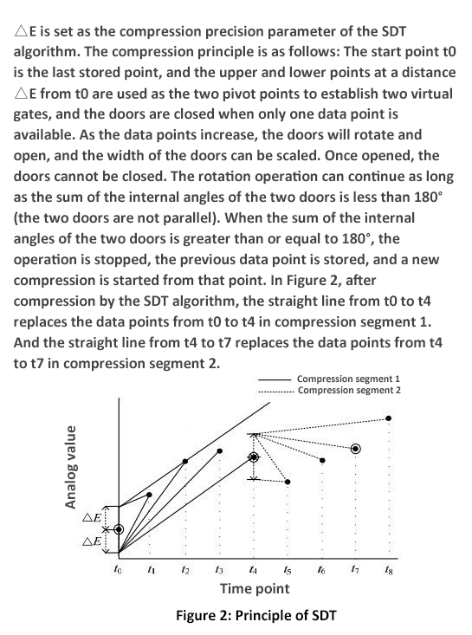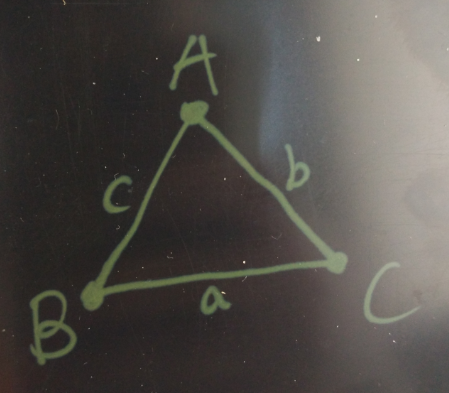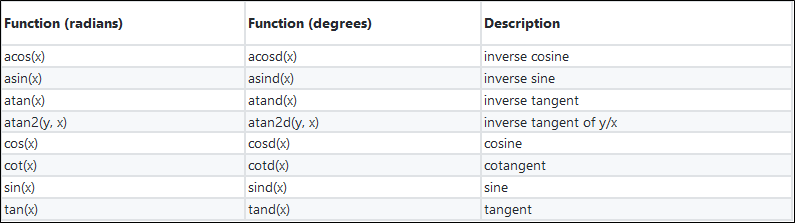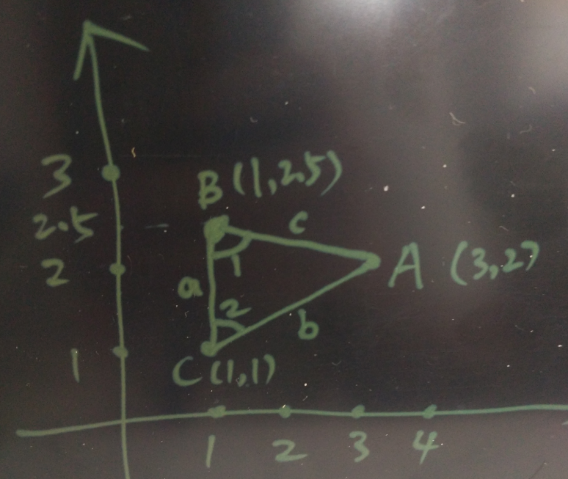# Community

Blog Events Webinars Tutorials Forum
×
Community Blog How You Can Use Trigonometric Functions in PostgreSQL to Obtain Further Insights

# How You Can Use Trigonometric Functions in PostgreSQL to Obtain Further Insights

This article discusses how PostgreSQL can use trigonometric functions to allow you to gain further insights about your data through these functions.

By Digoal

A few days ago, I wrote an article about the implementation of the SDT algorithm in PostgreSQL in which the ST_Azimuth function in PostGIS was used to compute the included angle. In reality, in PostgreSQL, you can also use trigonometric functions and three sides to compute the included angle. Consider the following figure:The method used in this article to compute the included angle is as follows:

``````      SELECT 180-ST_Azimuth(
ST_MakePoint(o_x, o_val+i_radius),    -- the point on the upper door
ST_MakePoint(v_x, v_val)              -- next point
)/(2*pi())*360 as degAz,                 -- the upper included angle
ST_Azimuth(
ST_MakePoint(o_x, o_val-i_radius),    -- the point on the lower door
ST_MakePoint(v_x, v_val)              -- next point
)/(2*pi())*360 As degAzrev               -- the lower included angle
INTO v_angle1, v_angle2;   ``````

## The Cosine theorem

I'm sure everyone remembers the Cosine theorem from Math class, which for us now can be summarized as: cosA=(b²+c²-a²)/(2bc). Well, this is also the basis of the discussion of this blog, too. For reference, consider the following figure:With this formula, the fixed points are A, B, and C, and the sides they are facing are a, b, and c, which are the unknown values. You can use the fixed point values to determine the unknown values.

## Trigonometric Functions in PostgreSQL

Here are some other trigonometric functions supported by PostgreSQL:## Example:

Consider this example. Three points are given: A(3,2), B(1,2.5), and C(1,1). Now you need to evaluate the included angles B and C. For reference, consider the figure below:Now, use the cosine formula.

``````cosB=(a²+c²-b²)/(2ac)

cosC=(b²+a²-c²)/(2ba)       ``````

First, calculate the length of the three sides.

``````postgres=# select point_distance(point(3,2), point(1,2.5)) as c , point_distance(point(3,2), point(1,1)) as b , point_distance(point(1,1), point(1,2.5)) as a;
c         |        b         |  a
------------------+------------------+-----
2.06155281280883 | 2.23606797749979 | 1.5
(1 row)    ``````

Then, perform the following operations:

``````cosB=(a²+c²-b²)/(2ac)
=(1.5^2 + 2.06155281280883^2 - 2.23606797749979^2) / (2*1.5*2.06155281280883)
=0.24253562503633260164

cosC=(b²+a²-c²)/(2ba)
=(1.5^2 + 2.23606797749979^2 - 2.06155281280883^2) / (2*2.23606797749979*1.5)
=0.44721359549995825124    ``````

Evaluate the degree of included angle 1.

``````postgres=# select acosd(0.24253562503633260164);
acosd
------------------
75.9637565320735
(1 row)  ``````

Evaluate the degree of included angle 2.

``````postgres=# select acosd(0.44721359549995825124);
acosd
-----------------
63.434948822922
(1 row)  ``````

The result is consistent with PostGIS results.

``````test=>  SELECT 180-ST_Azimuth(
ST_MakePoint(1,2.5),    -- the point on the upper door
ST_MakePoint(3,2)              -- next point
)/(2*pi())*360 as degAz,          -- the upper included angle
ST_Azimuth(
ST_MakePoint(1,1),      -- the point on the lower door
ST_MakePoint(3,2)              -- next point
)/(2*pi())*360 As degAzrev ;
degaz       |    degazrev
------------------+-----------------
75.9637565320735 | 63.434948822922
(1 row)  ``````

## The Source Code

Trigonometric functions belongs to functions for floating point operations.

``src/backend/utils/adt/float.c.``

Consider the following:

``````/*
*              acosd_q1                - returns the inverse cosine of x in degrees, for x in
*                                                the range [0, 1].  The result is an angle in the
*                                                first quadrant --- [0, 90] degrees.
*
*                                                For the 3 special case inputs (0, 0.5 and 1), this
*                                                function will return exact values (0, 60 and 90
*                                                degrees respectively).
*/
static double
acosd_q1(double x)
{
/*
* Stitch together inverse sine and cosine functions for the ranges [0,
* 0.5] and (0.5, 1].  Each expression below is guaranteed to return
* exactly 60 for x=0.5, so the result is a continuous monotonic function
* over the full range.
*/
if (x <= 0.5)
{
volatile float8 asin_x = asin(x);

return 90.0 - (asin_x / asin_0_5) * 30.0;
}
else
{
volatile float8 acos_x = acos(x);

return (acos_x / acos_0_5) * 60.0;
}
}

/*
*              dacosd                  - returns the arccos of arg1 (degrees)
*/
Datum
dacosd(PG_FUNCTION_ARGS)
{
float8          arg1 = PG_GETARG_FLOAT8(0);
float8          result;

/* Per the POSIX spec, return NaN if the input is NaN */
if (isnan(arg1))
PG_RETURN_FLOAT8(get_float8_nan());

INIT_DEGREE_CONSTANTS();

/*
* The principal branch of the inverse cosine function maps values in the
* range [-1, 1] to values in the range [0, 180], so we should reject any
* inputs outside that range and the result will always be finite.
*/
if (arg1 < -1.0 || arg1 > 1.0)
ereport(ERROR,
(errcode(ERRCODE_NUMERIC_VALUE_OUT_OF_RANGE),
errmsg("input is out of range")));

if (arg1 >= 0.0)
result = acosd_q1(arg1);
else
result = 90.0 + asind_q1(-arg1);

CHECKFLOATVAL(result, false, true);
PG_RETURN_FLOAT8(result);
}
man asin
NAME
asin, asinf, asinl - arc sine function

SYNOPSIS
#include <math.h>

double asin(double x);
float asinf(float x);
long double asinl(long double x);

///

CONFORMING TO
C99, POSIX. 1-2001.  The variant returning double also conforms to SVr4, 4.3BSD, C89.

acos(3), atan(3), atan2(3), casin(3), cos(3), sin(3), tan(3)  ``````

Now we need to functionalize this feature so that we can easily evaluate the degree of an included angle. To do so, run the following:

``````create or replace function angle (a point, b point, c point, d int) returns float8 as
\$\$

declare
ab float8 := point_distance(a, b);
ac float8 := point_distance(a, c);
bc float8 := point_distance(b, c);
cosa float8 := (ac^2 + ab^2 - bc^2) / (2*ac*ab);
cosb float8 := (bc^2 + ab^2 - ac^2) / (2*bc*ab);
cosc float8 := (ac^2 + bc^2 - ab^2) / (2*ac*bc);
begin
-- raise notice '%,%,%,  %,%,%', ab, ac, bc, cosa, cosb, cosc;
case d
when 1 then return acosd(cosa);  -- The first parameter point is the vertex of the included angle
when 2 then return acosd(cosb);  -- The second parameter point is the vertex of the included angle
when 3 then return acosd(cosc);  -- The third parameter point is the vertex of the included angle
else return null;
end case;
end;

\$\$
language plpgsql strict immutable;

postgres=# select angle(point(3,2), point(1,2.5), point(1,1), 1);
NOTICE:  00000: 2.06155281280883,2.23606797749979,1.5,  0.759256602365297,0.242535625036333,0.447213595499958
LOCATION:  exec_stmt_raise, pl_exec.c:3337
angle
------------------
40.6012946450045
(1 row)

postgres=# select angle(point(3,2), point(1,2.5), point(1,1), 2);
NOTICE:  00000: 2.06155281280883,2.23606797749979,1.5,  0.759256602365297,0.242535625036333,0.447213595499958
LOCATION:  exec_stmt_raise, pl_exec.c:3337
angle
------------------
75.9637565320735
(1 row)

postgres=# select angle(point(3,2), point(1,2.5), point(1,1), 3);
NOTICE:  00000: 2.06155281280883,2.23606797749979,1.5,  0.759256602365297,0.242535625036333,0.447213595499958
LOCATION:  exec_stmt_raise, pl_exec.c:3337
angle
-----------------
63.434948822922
(1 row)``````
0 0 0
Share on

# Related Products

• ##AnalyticDB for PostgreSQL

An online MPP warehousing service based on the Greenplum Database open source program

• ##AliwareMQ for IoT

A message service designed for IoT and mobile Internet (MI).

• ##IoT Platform

Provides secure and reliable communication between devices and the IoT Platform which allows you to manage a large number of devices on a single IoT Platform.

• ##ApsaraDB RDS for PostgreSQL

An on-demand database hosting service for PostgreSQL with automated monitoring, backup and disaster recovery capabilities